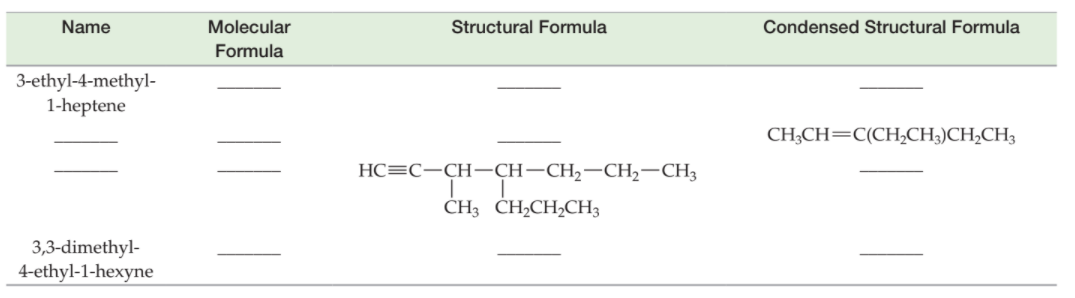×
Get Full Access to Introductory Chemistry - 5 Edition - Chapter 18 - Problem 64p
Get Full Access to Introductory Chemistry - 5 Edition - Chapter 18 - Problem 64p

×

# Solved: Complete the table.NameMolecular FormulaStructural FormulaCondensed StructuralISBN: 9780321910295 34

## Solution for problem 64P Chapter 18

Introductory Chemistry | 5th Edition

• Textbook Solutions
• 2901 Step-by-step solutions solved by professors and subject experts
• Get 24/7 help from StudySoup virtual teaching assistantsIntroductory Chemistry | 5th Edition

4 5 1 300 Reviews
21
0
Problem 64P

Complete the table.Step-by-Step Solution:

Solution 64P :

Step 1:

Here,we have to complete the given table :

 Name Molecular Formula Structural Formula Condensed Structural Formula 3-ethyl-4-methyl- 1-heptene _______ _______ _______ _______ _______ _______ CH3CH=C(CH2CH3)CH2CH3 _______ ______________ 3,3-dimethyl- 4-ethyl-1-hexyne _______ _______ _______

First, let’s consider the data in the 1st row :

Given :

Name : 3-ethyl-4-methyl-1-heptene.

Now, let’s write the structural formula :

There are 7 carbon atoms in the longest chain and the number 1 before heptene indicates the triple bond is on the 1st carbon atom. As per name, there is ethyl group on the 3rd carbon atom and methyl group on the 4th carbon atom. Hence the Structural formula for the given alkene is :Now, let’s write molecular formula :

We can derive the molecular formula looking at the structural formula of the alkene.

The molecular formula of the alkene is  : C10H20

Now, let’s write the condensed Structural Formula :

We can derive the condensed structural formula looking at the structural formula of the alkene.

The condensed structural formula of the alkene is  :

CH2CHCH(CH2CH3)CH(CH3)CH2CH2CH3

Step 2 of 5

Step 3 of 5

##### ISBN: 9780321910295

Unlock Textbook Solution